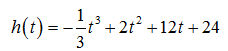# The altitude (in feet) attained by a model rocket t sec into flight is given by the function h(t). Find the maximum altitude attained by rocket. (round your answer to the nearest foot)h(t) = -1/3t^3 +2t^2 + 12 t +24 (t>=0)

Question
138 views

The altitude (in feet) attained by a model rocket t sec into flight is given by the function h(t). Find the maximum altitude attained by rocket. (round your answer to the nearest foot)

h(t) = -1/3t^3 +2t^2 + 12 t +24 (t>=0)

check_circle

Step 1

The altitude (in feet) attained by a model rocket t sec into flight is given by the functionStep 2

Firstly, we find h'(t) and solve h'(t)=0 for t

Step 3

Now, we find ...

### Want to see the full answer?

See Solution

#### Want to see this answer and more?

Solutions are written by subject experts who are available 24/7. Questions are typically answered within 1 hour.*

See Solution
*Response times may vary by subject and question.
Tagged in

### Derivative